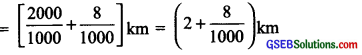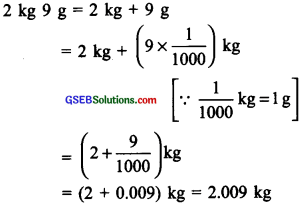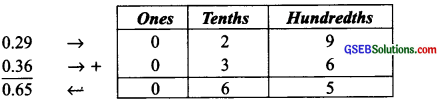# GSEB Solutions Class 6 Maths Chapter 8 Decimals InText Questions

Gujarat Board GSEB Textbook Solutions Class 6 Maths Chapter 8 Decimals InText Questions and Answers.

## Gujarat Board Textbook Solutions Class 6 Maths Chapter 8 Decimals InText Questions

Try These (Page 165)

Question 1.
Does canyon now write the following as decimals?Solution:
We have,
(i) 5 hundreds + 3 tens + 8 ones + 1 tenth
= 5 × 100 + 3 × 10 + 8 × 1 + 1 × $$\frac { 1 }{ 10 }$$
= 500 + 30 + 8 + $$\frac { 1 }{ 10 }$$
= 500 + $$\frac { 1 }{ 10 }$$ = 538.1

(ii) 2 hundreds + 7 tens + 3 ones + 4 tenths
= 2 × 100 + 7 × 10 + 3 × 1 + 4 × $$\frac { 1 }{ 10 }$$
= 200 + 70 + 3 + $$\frac { 4 }{ 10 }$$
= 273 + $$\frac { 4 }{ 10 }$$ = 273.4

(iii) 3 hundreds + 5 tens + 4 ones + 6 tenths
= 3 × 100 + 5 × 10 + 4 × 1 + 6 × $$\frac { 1 }{ 10 }$$
= 300 + 50 + 4 + $$\frac { 6 }{ 10 }$$
= 354 + $$\frac { 6 }{ 10 }$$ = 354.6Question 2.
Write the lengths of Ravi s and Raju’s pencils in ‘cm ’ using decimals. When Ravi and Raju measured the lengths of their pencils. Ravi s pencil was 7 cm and 5 mm long and Raju s pencil was 8 cm 3 mm long.
Solution:
We know that:
10 mm = 1 cm
Therefore, 1 mm = $$\frac { 1 }{ 10 }$$ cm
5 mm = 5 × $$\frac { 1 }{ 10 }$$ cm = $$\frac { 5 }{ 10 }$$ cm
and 3 mm = 3 × $$\frac { 1 }{ 10 }$$ cm = $$\frac { 3 }{ 10 }$$ cm
Now, 7 cm 5mm = 7 cm + 5 cm
= 7 cm + $$\frac { 5 }{ 10 }$$ cm
= 7.5 cm
Thus, lengths of Ravi’s pencil = 7.5 cm
Again,
8 cm 3 mm = 8 cm + 3 mm
= 8 cm + $$\frac { 3 }{ 10 }$$ cm
= 8.3 cm
Thus, length of Raju’s pencil = 8.3 cmQuestion 3.
Make three more examples similar to the one given in question 1 and solve them.
Solution:

Try These (Page 167)

Question 1.
Write $$\frac { 3 }{ 2 }$$ , $$\frac { 4 }{ 5 }$$ , $$\frac { 8 }{ 5 }$$ in decimal notation.
Solution:
(i) $$\frac { 3 }{ 2 }$$
We have, $$\frac { 3 }{ 2 }$$ = $$\frac{3 \times 5}{2 \times 5}$$ = $$\frac { 15 }{ 10 }$$ = 1.5
∴ $$\frac { 3 }{ 2 }$$ = 1.5

(ii) $$\frac { 4 }{ 5 }$$
We have, $$\frac { 4 }{ 5 }$$ = $$\frac{4 \times 2}{5 \times 2}$$ = $$\frac { 8 }{ 10 }$$ = 0.8
= $$\frac { 8 }{ 10 }$$ = 0.8
Thus, $$\frac { 4 }{ 5 }$$ = 0.8

(iii) $$\frac { 8 }{ 5 }$$
We have, $$\frac { 8 }{ 5 }$$ = $$\frac{8 \times 2}{5 \times 2}$$ = $$\frac { 16 }{ 10 }$$ = 1.6
Thus, $$\frac { 8 }{ 5 }$$ = 1.6

Try These (Page 175)

Question 1.
(i) Write 2 rupees 5 paise and 2 rupees 50 paise in decimals.
(ii) Write 20 rupees 7 paise and 21 rupees 75 paise in decimals.
Solution:
(i) (a) 2 rupees 5 paise:
2 rupees + 5 paise = 2 rupees + $$\frac { 5 }{ 10 }$$ rupees= (2 + 0.05) rupees = ₹ 2.05

(b) 2 rupees 50 paise:
2 rupees + 50 paise = 2 rupees + $$\frac { 50 }{ 100 }$$ rupees= (2 + 0.50) rupees = ₹ 2.50

(ii) (a) 20 rupees 7 paise:
20 rupees + 7 paise
= 20 rupees + 7 × $$\frac { 1 }{ 100 }$$ rupess= 20 rupees + 7 paise
= ₹ (20 + 0.07) = ₹ 20.07

(b) 21 rupees 75 paise:
21 rupees + 75 paise
= 21 rupees + 75 x $$\frac { 1 }{ 100 }$$ rupees
= 21 rupees + $$\frac { 75 }{ 100 }$$ rupees
= ₹ [21 + 0.75] = ₹ 21.75

Try These (page 176)

Question 1.
Can you write 4 mm in ‘cm’ using decimals?
Solution:
Yes,
Since 10 mm = 1 cm
∴ 1 mm = $$\frac { 1 }{ 10 }$$ cm
or 4 mm = 4 × $$\frac { 1 }{ 10 }$$ cm = 0.4 cmQuestion 2.
How will you write 7 cm 5 mm in ‘cm’ using decimals?
Solution:
7 cm 5 mm:
Since, 10 mm = 1 cm
Now, 7 cm 5 mm = 7 cm + 5 mm
= 7 cm + 5 × $$\frac { 1 }{ 10 }$$ cm =cm
= (7 + 0.5) cm = 7.5 cmQuestion 3.
Can you now write 52 m as ‘km’ using decimals? How will you write 340m as ‘km’ using decimals? how will you write 2008 m in ‘km’?
Solution:
Yes, we can change the given ‘meters’ into kilometers.
(a) 52 m
∵ 1000 m = 1 m
∴ 1 m = $$\frac { 1 }{ 1000 }$$ km
or 52 m = 52 × $$\frac { 1 }{ 1000 }$$ km = 0.052 km

(b) 340 m
∵ 1 m = $$\frac { 1 }{ 1000 }$$ km
∴ 340 m = 340 × $$\frac { 1 }{ 1000 }$$ km
= $$\frac { 340 }{ 1000 }$$ km = 0.340 km

(c) 2008 m
∵ 1 m = $$\frac { 1 }{ 1000 }$$ km
∴ 2008 m = 2008 × $$\frac { 1 }{ 1000 }$$ km = $$\frac { 2008 }{ 1000 }$$ km= (2 + 0.008) km = 2.008 km

Try These (page 176)

Question 1.
Can you now write 456 g as ‘kg’ using decimals?
Solution:
Since 1000 g = 1 kg
∴ 1 g = $$\frac { 1 }{ 1000 }$$ kg
∴ 456 g = $$\frac { 1 }{ 1000 }$$ x 456 kg
= $$\frac { 456 }{ 1000 }$$ kg = 0.456 kgQuestion 2.
How will you write 2 kg 9 g in ‘kg’ using decimals?
Solution:Try These (page 178)

Question 1.
Find
(i) 0.29 + 0.36
(ii) 0.7 + 0.08
(iii) 1.54 + 1.80
(iv) 2.66 + 1.85
Solution:
(i) 0.29 + 0.36∵ (9 + 6) hundredths = 15 hundredths = 1 tenths + 5 hundredths
Thus, 0.29 + 0.36 = 0.65

(ii) 0.7 + 0.08Thus, 0.7 + 0.08 = 0.78

(iii) 1.54 + 1.80Thus, 1.54 + 1.80 = 3.34
Note: ∵ 5 tenths + 8 tenths = 13 tenths and 13 tenths = 10 tenths + 3 tenths = 1 one + 3 tenths

(iv) 2.66 + 1.85Thus, 2.66 + 1.85 = 4.51

Try These (page 180)

Question 1.
Subtract 1.85 from 5.46
Solution:
1.85 and 5.46 are ‘like decimals’.Here, 1 is borrowed from ‘ones’ and given to tenths such that:
4 tenths + 10 tenths = 14 tenths
5 ones – 1 one = 4 onesQuestion 2.
Subtract 5.25 from 8.28.
Solution:
5.25 and 8.28 are ‘like decimals’.Question 3.
Subtract 0.95 from 2.29.
Solution:
0.95 and 2.29 are ‘like decimals’.Question 4.
Subtract 2.25 from 5.68
Solution:
2.25 and 5.68 are ‘ like decimals’.